Students can download 4th Maths Term 1 Chapter 4 Measurements Ex 4.5 Questions and Answers, Notes, Samacheer Kalvi 4th Maths Guide Pdf helps you to revise the complete Tamilnadu State Board New Syllabus, helps students complete homework assignments and to score high marks in board exams.

## Tamilnadu Samacheer Kalvi 4th Maths Solutions Term 1 Chapter 4 Measurements Ex 4.5

Question 1.
Convert into cm
(a) 5m
(b) 7m
(c) 9m
(d) 16m
(a) 5m
5m = 5 × 100 = 500 cm

(b) 7m
7m = 7 × 100 = 700 cm(c) 9m
9m = 9 × 100 = 900 cm

(d) 16m
16m = 16 × 100 = 1600 cm

Question 2.
Convert into m
(a) 6000 cm
(b) 4000 cm
(c) 13000 cm
(d) 17000 cm
(a) 6000 cm
6000 cm = 6000 ÷ 100 = 60 m

(b) 4000 cm
4000 cm = 4000 ÷ 100 = 40 m

(c) 13000 cm
13000 cm = 13000 ÷ 100 = 130 m

(d) 17000 cm
17000 cm = 17000 ÷ 100 = 170 mQuestion 3.
(a)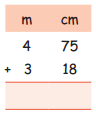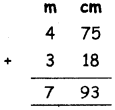4m 75cm + 3m 18cm = 7m 93cm

(b)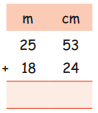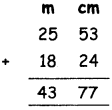25m 53cm + 18m 24cm = 43m 77cm(c)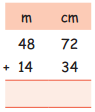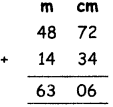48m 72cm + 14m 34cm = 63m 06cm

Question 4.
Subtract
(a)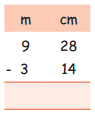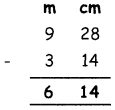9m 28cm – 3m 14cm = 6m 14cm(b)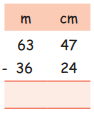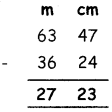63m 47cm – 36m 24cm = 27m 23cm

(c)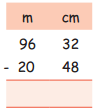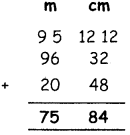96m 32cm – 20m 48cm = 75m 84cmQuestion 5.
Raju used 13 m 25 cm ribbon for making his project. If he had bought 20 m of ribbon, How much ribbon is left with him?
Total length of ribbon = 20 m
Raju used = 13 m 25 cm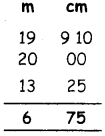Remaining length of ribbon = 6 m 75 cm

Question 6.
The distance between bus stand and school is 81 m 40 cm and the distance between school and temple is 20 m 10 cm. What is the total distance from bus stand to temple ?
Distance between bus stand to school = 81 m 40 cm
Distance between school to temple = 20 m 10 cm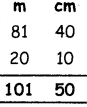Distance between bus stand to temple is = 101 m 50 cmQuestion 7.
Arul has a 4 metre long piece of wood. He wants to cut it into 2 equal lengths. How long should each piece be in millimetres?